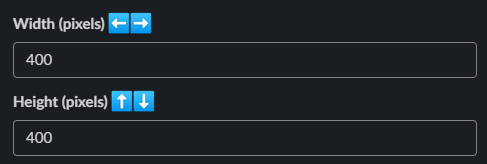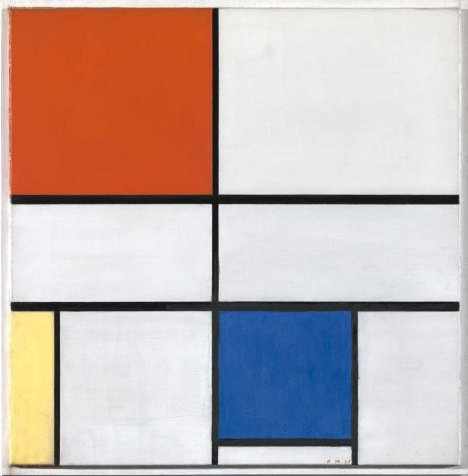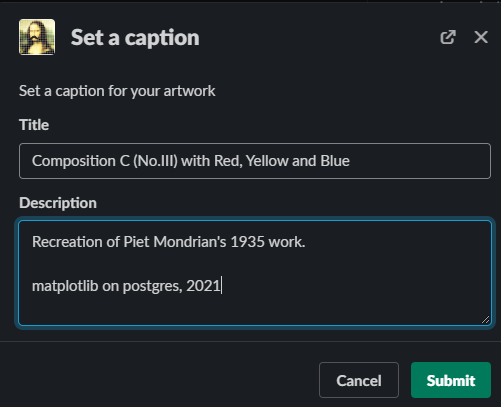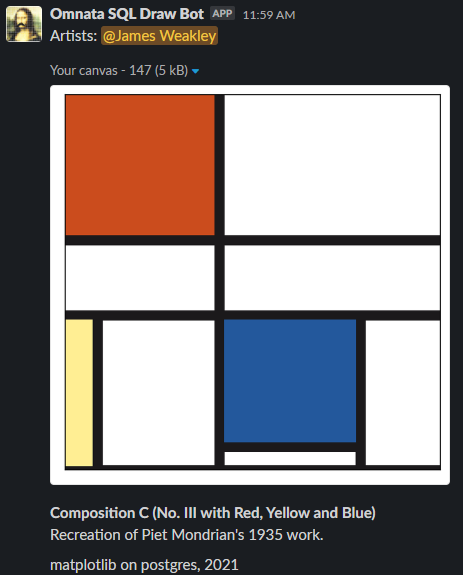# A larger canvas 🎨

Now that we understand what's going on at the pixel level, let's zoom out a bit and create a real artwork.

Let's create a `400`x`400` white canvas.

Hit Submit, and wait for the canvas to arrive.# What to draw?

So that we can get some practise drawing basic shapes, let's see if we can recreate Piet Mondrian's Composition C (No. III with Red, Yellow and Blue)We can actually do this with a single `CASE` statement!

First, I grabbed the colour hex codes from the original by using this chrome extension.

Then the SQL:

update bitmap_pixels set colour = case
-- red square
when x < 160 and y > 250 then '#CB4C1D'
-- blue square
when x between 170 and 310 and y between 30 and 160 then '#23589C'
-- yellow rectangle
when x < 30 and y between 5 and 160 then '#FFEE93'
-- black lines, horizontal
when y between 240 and 250 then '#1B191B'
when y between 160 and 170 then '#1B191B'
when y <= 5 then '#1B191B'
when x between 170 and 310 and y between 20 and 30 then '#1B191B'
-- black lines, vertical
when x between 160 and 170 and y > 5 then '#1B191B'
when y between 5 and 160 and (x between 30 and 40 or x between 310 and 320) then '#1B191B'
-- white squares
else '#ffffff'
end# A better way

This is cool, but it'll be hard to move on from Mondrian if we can only target x or y ranges.
Did you know that Postgres has built-in geometric functions? Let's redraw our artwork using these.

For example, we can draw our red square using the '<@' operator, by asking whether the x,y point is contained within a box that has bottom left coordinates (0,250) and top right (160,400). So:
`when x < 160 and y > 250 then '#CB4C1D'`
becomes
`when point(x,y) <@ box '((0,250),(160,400))' then '#CB4C1D'`

Similarly, to draw the long vertical black line, we can use the '<->' operator to ask whether the x,y point is within a 5px distance of a line with starting point (165,400) and ending at point (165,5). So:
`when x between 160 and 170 and y > 5 then '#1B191B'`
becomes
`when point(x,y) <-> lseg'((165,400),(165,5))' < 5 then '#1B191B'`

# Over to you!

Why don't you try re-drawing Mondrian's using only geometric functions? Then for bonus marks, add some your own touches! You could draw circles, triangles, or any type of polygon!

Geometry is the foundation of SQL draw, so it's worth getting some practise in on different shapes!

Once you've made it your own, don't forget to set a caption using the "Set caption" message shortcut: